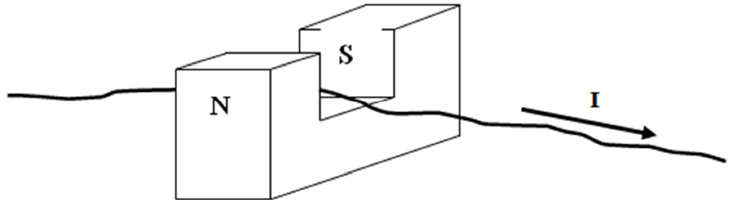# The Motor Effect

This is part of the HSC Physics course under the topic The Motor Effect

### HSC Physics Syllabus

• investigate qualitatively and quantitatively the interaction between a current-carrying conductor and a uniform magnetic field F=lI_{_|_}B=lIB\sin\theta to establish: (ACSPH080, ACSPH081)
–conditions under which the maximum force is produced
–the relationship between the directions of the force, magnetic field strength and current
–conditions under which no force is produced on the conductor

### What is the Motor Effect?

This video will explain what the motor effect is. It will qualitatively and quantitatively investigate the interaction between a current-carrying conductor and a uniform magnetic field.

### The Motor Effect

The motor effect refers to the phenomenon where a current-carrying conductor experiences a force in an external magnetic field. The direction of the force is determined by the right hand palm rule:

• Thumb points in direction of conventional current (against flow of electrons)
• Fingers point in the direction of magnetic field lines
• Palm faces the direction of force

### Quantitatively Deriving the Motor Effect Equations

Recall that a moving charged particle experiences a force in an external magnetic field. A current in a conductor experiences a force via the same mechanism since a current is moving electrons. Hence

$$F=qvB\sin{\theta}$$

Since current is defined as charge over time, we let I=q/t, so q=Ixxt. Velocity is displacement over time, so we let v=s/t=l/t by taking the length of the conductor to be the displacement. Substituting these gives,

$$F=({I\times{t}})({\frac{l}{t}})B\sin{\theta}$$

$$F=lIB\sin{\theta}$$

where,

• B is the magnetic field strength (T or Wb m-2
• I is the current (A)
• l is the length of the current-carrying conductor within the magnetic field (m)
• \theta is the angle between the conductor and magnetic field lines

From this equation, we deduce that:

• maximum force occurs when the conductor is perpendicular (\theta= 90º) to the magnetic field lines
• minimum force (zero) occurs when the conductor is parallel (\theta= 0º) to the magnetic field lines

### Investigating the Motor Effect

A single loop of wire is placed within a horse-shoe magnet and connected to an external battery.The direction of current is determined by how the wired is connected to the battery.

When the battery is switched on, the wire moves either upwards or downwards due to the motor force. For example, in the diagram above: the wire moves upwards when the battery is switched on.

The magnitude of the force experienced by the wire depends on:

• strength of magnetic field B produced by the horse-shoe magnet
• length of wire that is inside the magnetic field
• magnitude of current
• angle between current direction and the magnetic field lines (in your investigation, this is usually set to 90º to maximise the resultant force. Greater force leads to a greater extent of wire movement.

﻿Previous section: Comparing Charged Particles in Magnetic Fields to Electric Fields